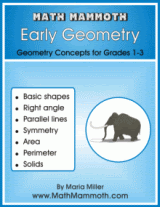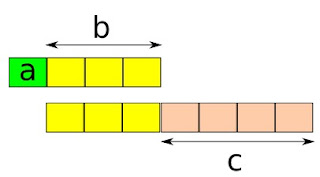## Posts

Showing posts from September, 2008

### Another algebra problem - or is algebra needed?

Updated!

There are some marbles in Box A and Box B. If 50 marbles from Box A and 25 from Box B are removed each time, there will be 600 marbles left in Box A when all marbles are removed from Box B. If 25 marbles from Box A and 50 marbles from Box B are removed each time, there will be 1800 marbles left in Box A when all marbles are removed from Box B. How many marbles are there in each box?

Again, this is from Singapore and teachers have told students not to use algebra to solve this question. However, any form of heuristic tools are allowed to facilitate the students in solving the questions.

I'd like to point out that I feel it's a good problem, but students might benefit from some "preparation". You could set up a preparation problem like this:

Jar A has 100 marbles and jar B has 40 marbles. You will start removing marbles one by one from jar A, but by 2's from jar B. How many marbles are left in jar A when jar B is empty?
What if you remove 2 marbles at a time fr…

### An algebra problem

This question was set in one of the renowned primary school from Singapore. Given to me by "anonymous" to solve.
Andy has \$200 more than Peter. Andy gives 60% of his money to Peter. Peter then gives 25% of his money to Andy. In the end, Peter has \$200 more than Andy. How much did Andy have at first?This is a great problem to solve with algebra. Why don't you try it first, before reading further? It sounds kind of interesting... first one guy has \$200 more than the other, and in the end it's reversed.

Solution:

Let A be the initial amount Andy has, and P the initial amount Peter has.
Then we know that A = P + 200. We're going to use that later, but for now I'm going to write it all in terms of A and P.

Andy gives 60% of his money or 0.6A to Peter.

Peter has now P + 0.6A.
Andy has now 0.4A.

Peter then gives 25% of his money to Andy. But this isn't 0.25P because Peter doesn't have P dollars anymore because Andy already gave him some. It's 0.25 (P + 0.6A) th…

This free lesson about mean and mode will get you a foretaste for my upcoming 5-A Complete Curriculum from the LightBlue Series.

Mean, Mode, and Bar Graphs - lesson for 5th grade.

In the lesson I highlight the idea of mode versus mean (average) and when you can calculate the mean. Students also graph the data in bar graphs.

I didn't include the median because elementary lessons on mean, median, and mode tend to concentrate on the calculation aspect only, and I didn't want that. In this lesson they at least get to graph the data and think if mean (average) is "calculable". So I decided to postpone the median till 6th grade...

But here are some other lessons on these topics. Even with these you can see how much the actual calculations dominate the lessons.

Using and Handling Data
Simple explanations for finding mean, median, or mode.
www.mathsisfun.com/probability

Mode of a Set of Data

A very simple and clear lesson with examples and interactive quiz questions…

### A quite long prime

Mathematicians at UCLA have verified now the largest known prime number, 13 million digits long!

It's a Mersenne prime, in the form 2p − 1. This new prime is 243,112,609 − 1. (And no, you can't put it in your calculator and get an answer... )

This kind of prime hunting requires a network of computers running together to do the massive amounts of calculations.

### Credit card math

Considering the crisis taking place within U.S. financial institutions, it's a good reminder of how we MUST teach our kids how to work out the math with credit cards, mortgages, and any type of loans.

Murray Bourne from squareCircleZ has taken this matter to heart and has written a good lesson about math that goes into taking a loan. He's also analyzed several misleading credit card ads and figured out the TRUE interest rate (which you find out after reading the fine print).

First go here:

Credit Cards - a simplified discussion on how banks make money on credit cards. This is a must read for all people (young or old) who even consider taking a credit.

Then check these posts and warn your youngsters:

Misleading Credit Card Advertising. This ad advertises "a attractive interest rate of 5% p.a." (p.a. means per annum or yearly interest rate), but has a "small" administration fee of 6%.

### Two new books in the Blue Series64 pages
(of which 37 are lesson pages)

Sample pages (PDF)

Contents and Introduction
Shapes
Right Angles
Line Symmetry
Perimeter
Solids 1. Math Mammoth Early Geometry covers geometry topics for the early elementary grades (approximately grades 1-3).

The first lessons in this book have to do with shapes - that is where geometry starts. Children learn the names of the common shapes, and also put several shapes together to form new ones, or divide an existing shape into new ones. They practice using a ruler to draw various shapes and are introduced to tilings.

Next children learn the concepts of parallel lines and lines that are at a right angle (perpendicular lines). The book also has beginner lessons about symmetry, area, perimeter, and solids.

After studying these early geometry lessons, you can continue the study of geometry with Math Mammoth Geometry 1 book. In it, children will learn to classify figures (during grades 4-6) according to their …

### A simple ratio problemProblem: If a:b = 1:3 and b:c = 3:4, find a:c.

Two ratios are given, third is to be found. This is very very simple. The picture shows the two given ratios as blocks.

We can see that a is one block and c is four blocks, so the ratio a:c is 1:4.

You don't need an image for that, of course, since the original ratios are so easy. If a:b=1:3 and b:c=3:4, b being the same in both cases, we can write the ratio a:b:c as 1:3:4 right off.

But what if the numbers weren't so friendly? What if it said this way:

If a:b = 1:3 and b:c = 5:7, find a:c.

This is solvable in various ways. I'll use equivalent ratios, in other words change the given ratios to equivalent ratios until we find ones where the b's are the same.

In the first ratio, 1:3, b is 3. In the other ratio, 5:7, it is 5. We can make those to be 15 by changing the ratios to equivalent ratios - which is done in an identical manner as changing fractions to equivalent fractions.

1:3 = 5:15 and 5:7 = 15:21.

Now the ratio of a:b:c is …

These are just some links sent my way over the last few months that I thought you might enjoy.
Teach Banzai is an online system for financial education. It sounds really interesting! Students can set up various "jars" (budgets), enter transactions (real or fictional), and continue using the system for life if they so wish. It's all free and there are lesson plans offered.
SpaceTime TV
Watch educational videos online from full length documentaries to short video clips featuring shows from PBS, National Geographic, and the History Channel. They've done a good job of collecting the best free science and history videos. I can just imagine how difficult and time-consuming it'd be to find those if you went searching on YouTube an other video sites yourself.

Dimensions
Interesting movie - which is available totally free online - about how mathematicians can visualize the fourth dimension. Some of that stuff will give you "mathematical vertigo", but some of the chap…

### Carnival of Homeschooling

Carnival of Homeschooling number 141 is now online at Why Homeschool. Go visit and read it! My submission was the review I recently did of Danica McKellar's books.

Here's one pick from the carnival I enjoyed: Hands-on ABC Order Activities which has some neat ideas for helping kids learn their ABC order.

### A word problem

Another word problem was sent my way.
Todd, Chris, and Rod have 30 birds. Rod has five times as many as Todd. Todd has one fourth the number Chris has. How many birds do they each have?The numbers in this problem are so small that I won't make any equations -- it might be QUICKER to use guess and check!

Just notice Todd has the least amount, and once you know (or guess) how many birds Todd has, then you immediately know how many Chris and Rod have. Rod will have 5 times the amount Todd has, and Chris will have four times the amount Todd has.

We will start by giving Todd 1 bird, and checking if the condition is met that the total becomes 30. Then we will give Todd 2 birds and check again.

Rod 5 10 15
Chris 4 8 12
Todd 1 2 3
---------------------
Total 10 20 30

So Todd has 3 birds, Chris has 12, and Rod has 15.

I'm guessing this might be a problem from algebra course, and that the author/teacher intends algebra to be used. But I would say that use the most…

### Abacus and basic division concept

Let's be reminded first of all that there are two basic "interpretations" of division:

1) Sharing division. In it, you think that 16 / 2 means "If there are 16 beads divided between 2 people, how many does each one get?

2) Quotative division. In it, you think how many groups of the same size you can form. Or, "how many times does the divisor fit into the dividend?" For example, 16 / 2 is interpreted as: "If you have 16 beads, how many groups of 2 beads can you make?"

Sharing division is easy to understand as a concept, but it's hard to do without the knowledge of multiplication tables. Just imagine, if a child who doesn't know their times tables is trying to find the answer to the question, "If there are 56 strawberries and 8 people, how many will each one get?" It'll take some guesswork, trying and checking.

But quotative division is easy for children to do with the help of manipulatives (or by drawing pictures). Just make a g…

### Timed drills for math facts?

Are timed math tests (drills) necessary and for what purpose? If necessary, how often should facts be tested? Are timed math tests recommended for students who have "math issues." , such as low confidence and slower progress. I simply feel that timed drills are a tool among many, when it comes to learning math facts. Some kids will "thrive" on them, or in other words quickly learn when they are used. Perhaps they like racing against the clock or like the challenge. There exist computer games that are timed that can work very well for drilling facts. Check for example:

Math Magician games has a simple 2-minute countdown, and if you answer 20 questions in that time, you get an award.

Some of the games at Sheppard Software don't time you but give you more points the faster you go. That site is actually filled with several types of games just for math facts practice.

Yet for other kids timed drills may be counterproductive and end up in tears and frustration. The …